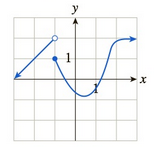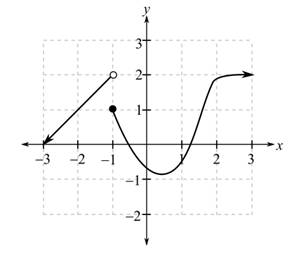Chapter 10.1, Problem 41EFinite Mathematics and Applied Cal...

7th Edition
Stefan Waner + 1 other
ISBN: 9781337274203

Solutions

Chapter
SectionFinite Mathematics and Applied Cal...

7th Edition
Stefan Waner + 1 other
ISBN: 9781337274203
Textbook Problem

In Exercises 35–48 the graph of f is given. Use the graph to compute the quantities asked for. [HINT: See Examples 4–5.]a. lim x → − 2 f ( x ) b. lim x → 1 + f ( x ) c. lim x → 1 − f ( x ) d. lim x → − 1 f ( x ) e. f ( − 1 ) f. lim x → + ∞ f ( x )(a)

To determine

The value of limx2f(x) using the graph which is as follows:Explanation

Given information:

The graph is as follows:

From the graph, evaluate the limit for x tends to 2 for the function f(x).

For this, take a point on the graph to the left of x=2 and move along the curve, so that the x-coordinate approaches 2. Then, it is noticed from the graph that the y-coordinate approaches 1

(b)

To determine

The value of limx1+f(x) using the graph which is as follows:(c)

To determine

The value of limx1f(x) using the graph which is as follows:(d)

To determine

The value of limx1f(x) using the graph which is as follows:(e)

To determine

The value of f(1) using the graph which is as follows:(f)

To determine

The value of limx+f(x) using the graph which is as follows:Still sussing out bartleby?

Check out a sample textbook solution.

See a sample solution

The Solution to Your Study Problems

Bartleby provides explanations to thousands of textbook problems written by our experts, many with advanced degrees!

Get Started

Find more solutions based on key concepts